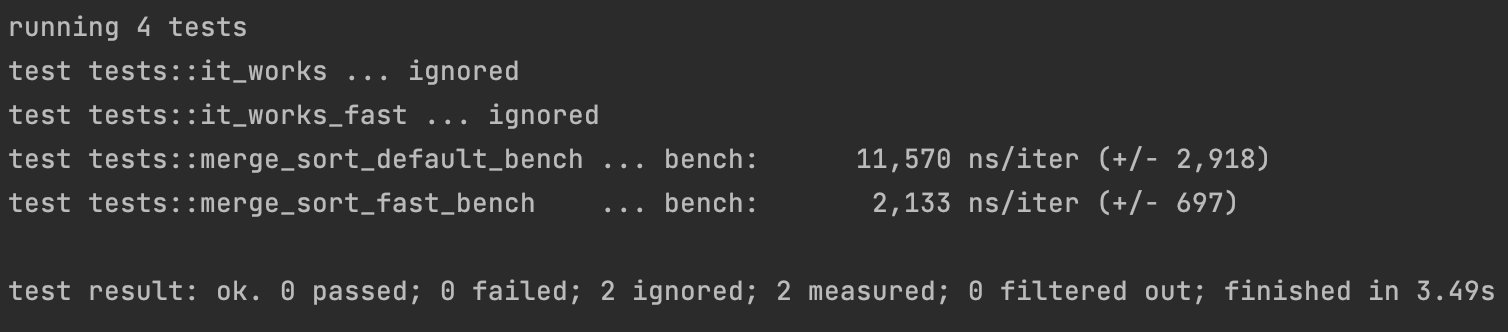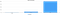# Speeding up algorithms with arena allocators

Choosing the best algorithms is very important, but how we can speed up the already chosen algorithms?spoilers

Today, I will show you how we can speed up the mergesort algorithm more than five times on 100 elements in an array with the arena allocator.

# Introduction

In this chapter, we will talk about minimal theory, which you need to understand before we will talk about arena allocators.

## Memory allocation

Almost every step in your code needs some memory. When we need to save some state, we need to ask for memory from OS. For doing that we have the two ways:

1) Static memory allocation — allocated by the compiler. The exact size and type of memory must be known at compile time.

2) Dynamic memory allocation — memory allocated during run time. Exact sizes or amounts (like the size of an array, for example) do not have to be known by the compiler in advance. This memory is allocated by the run time system. To allocate memory dynamically, we have to use pointers.

More about allocations you can read here.

So, when you creating some variable (like vector or string) in most cases you will call mmap, brk, or something like this for allocating memory. All of these functions ask OS for memory and wait for a response. Using these functions called system calls and most of them are really slow.

## CPU caches

CPU caches is a smaller, faster memory, located closer to a processor core, which stores copies of the data from frequently used main memory locations.Less is faster

As we see, access data in the L1 cache faster than RAM one hundred times!

But how data go to the CPU caches?

When the processor needs to read or write a location in memory, it first checks for a corresponding entry in the cache. The cache checks for the contents of the requested memory location in any cache lines that might contain that address. If the processor finds that the memory location is in the cache, a cache hit has occurred. However, if the processor does not find the memory location in the cache, a cache miss has occurred. In the case of a cache hit, the processor immediately reads or writes the data in the cache line. For a cache miss, the cache allocates a new entry and copies data from the main memory, then the request is fulfilled from the contents of the cache.

More about that you can read on Wiki.

# Arena allocator

There I will answer the three questions:

• How it’s work?
• How we can use that?
• What are the limitations?

## Memory access problem

When you use some variables in a code (or structures like a linked list), you could not guaranteed memory location. Your algorithm can works when one variable store in one cache line and after that a code needs another variable from another cache line. It leads to cache misses and asking RAM which 100 times slower.

## Solution

We can allocate a big memory space for your algorithm and store your variables in this space. Thanks to that your variables will be located nearby. It will increase cache hits on CPU caches and may speed up your code execution in hundred times! This is a solution called the arena (also called region-based, area) allocator.

The simple realization of arena allocator is:

`void* chunk = malloc(9999); // our arena allocator will be able to allocate 9999 bytessize_t current_align = 0;void* arena_alloc(size_t n) { current += n; return chunk + current_align - n; } // arena_alloc will be replaced malloc for our algorithm`

The wiki article about arena allocators.

## Limitations

The main limitation of an arena allocator is that you can free only fully allocated memory space.

Also, your code can overflow memory, available in the allocator. So, you need to use something like a linked list with chunks of allocated memory for solving that.

# Speed up merge sort algorithm in Rust

For example, let’s try to optimize the simple mergesort realization:

`pub fn merge_sort<T: Clone + PartialOrd>(x: &mut [T]) {    let n = x.len();    let m = n / 2;    if n <= 1 {        return;    }    merge_sort(&mut x[0..m]);    merge_sort(&mut x[m..n]);    let mut y: Vec<T> = x.to_vec();    merge(&x[0..m], &x[m..n], &mut y[..]);    x.clone_from_slice(&y);}fn merge<T: Clone + PartialOrd>(x1: &[T], x2: &[T], y: &mut [T]) {    assert_eq!(x1.len() + x2.len(), y.len());    let mut i = 0;    let mut j = 0;    let mut k = 0;    while i < x1.len() && j < x2.len() {        if x1[i] < x2[j] {            y[k] = x1[i].clone();            k += 1;            i += 1;        } else {            y[k] = x2[j].clone();            k += 1;            j += 1;        }    }    if i < x1.len() {        y[k..].clone_from_slice(&x1[i..]);    }    if j < x2.len() {        y[k..].clone_from_slice(&x2[j..]);    }}`

Let’s write a benchmark:

`#[bench]fn merge_sort_fast_bench(b: &mut Bencher) {    b.iter(|| {        let mut arr = Vec::from(SORT_ARR); // Sort array it's randomly generated array with 100 numbers        merge_sort(&mut arr);    });}`

On 100 elements this algorithm in average longs with 11,000 ns.

So, how it will be work with an arena allocator? We will use the bumpalo library.

`pub fn merge_sort_fast<T: Clone + PartialOrd>(x: &mut [T]) {    let bump = Bump::new(); // create our arena allocator    merge_sort_fast_realization(x, &bump);}fn merge_sort_fast_realization<T: Clone + PartialOrd>(x: &mut [T], allocator: &Bump) {    let n = x.len();    let m = n / 2;    if n <= 1 {        return;    }    let (left, right) = x.split_at_mut(m);    merge_sort_fast_realization(left, allocator);    merge_sort_fast_realization(right, allocator);    let y: &mut [T] = allocator.alloc_slice_clone(x);    let (left, right) = x.split_at(m);    merge(left, right, y);    x.clone_from_slice(&y);}fn merge<T: Clone + PartialOrd>(x1: &[T], x2: &[T], y: &mut [T]) {    assert_eq!(x1.len() + x2.len(), y.len());    let mut i = 0;    let mut j = 0;    let mut k = 0;    while i < x1.len() && j < x2.len() {        if x1[i] < x2[j] {            y[k] = x1[i].clone();            k += 1;            i += 1;        } else {            y[k] = x2[j].clone();            k += 1;            j += 1;        }    }    if i < x1.len() {        y[k..].clone_from_slice(&x1[i..]);    }    if j < x2.len() {        y[k..].clone_from_slice(&x2[j..]);    }}`

And the benchmark:

`#[bench]fn merge_sort_fast_bench(b: &mut Bencher) {    b.iter(|| {        let mut arr = Vec::from(SORT_ARR);        merge_sort_fast(&mut arr);    });}`

Also, I write the benchmark with standard sorting:

`#[bench]fn sort_standard_bench(b: &mut Bencher) {    b.iter(|| {        let mut arr = Vec::from(SORT_ARR);        arr.sort_unstable()    });}`

Let’s see the results:

`running 5 teststest tests::it_works ... ignoredtest tests::it_works_fast ... ignoredtest tests::merge_sort_default_bench  ... bench:      11,511 ns/iter (+/- 1,399)test tests::merge_sort_fast_bench     ... bench:       2,157 ns/iter (+/- 524)test tests::sort_standard_bench       ... bench:         162 ns/iter (+/- 45)`

So, better to use standard realization…

# Conclusion

Arena allocators may really speed up your algorithms, but it will work fine only when you already chose a good algorithm. The bubble sort will work slower than other fast sorting algorithms even with an arena allocator.

But when you need to increase performance, using arena allocators is a very good idea!

Software Developer from VK

## More from Mikhail Panfilov

Software Developer from VK